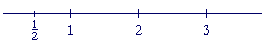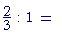The Evolution of the

R E A L  N U M B E R S

5

# FRACTIONS

TO MEASURE something requires the name of a number. But the names of the natural numbers are not sufficient, because what we measure will not always be a multiple of the unit of measure, that is, of 1. In order to measure we have to create numbers that are parts of 1. Those are the fractions.

What is more, in a natural number, the units are indivisible. We therefore must extend the idea of a "number" so that it corresponds to things that we measure, that is, things that are continuous.

Let 1 therefore now be the measure of a continuous unit:  1 inch,1 meter, 1 hour; and let us imagine that we have to invent a numeral -- a symbol, a "number" -- for half of 1.

What symbol should we invent?

 The symbol invented, of course, is 1 over 2: 12 .

But why?

Because of the ratio of 1 to 2.  Since 1 is one half of 2, then the number we write

 in this way, 12 ,  is one half of 1.  We call it the number

"one-half."

In fact, we know a number according to how it is related to 1.What is our understanding of  "2"?  It is twice as much as 1.  What is "3"? It is three times 1. And the number we write

 as " 12 " is one half of 1.

We know every number according to its ratio to 1, which is the source.

A fraction that is less than 1 we call a proper fraction.  And in English, the proper fractions have the same names as the ratio of the numerator to the denominator.

 This number 23 is called "two-thirds" because of the

ratio of 2 to 3.  2 is two thirds of 3.  That fraction, moreover, is the measure of the length that has the same ratio to 1:23 is to 1  as  2 is to 3.

The fraction called "two-thirds" is two thirds of 1.

(Notice that we write the name of the fraction hyphenated, but not the name of the ratio.  In that way we maintain the distinction between fractions and ratios.  A fraction is a number; a ratio is a relationship between numbers and between lengths.)

The same ratio to 1

Every fraction has a ratio to 1: the same ratio that the numerator has to the denominator.

 ab : 1  =  a : b

Problem 1.   a)  What number is the fourth part of 1?  Write its symbol and also write its name in words.

To see the answer, pass your mouse over the colored area.
To cover the answer again, click "Refresh" ("Reload").

 14 .  One-fourth.

b)  When we speak of a fourth of 1, does '1' refer to a continuous unit or
b)  discrete?

Continuous.

 c)  Place 14 on this number line.Problem 2.   Why is this number 25 called "two-fifths"?

Because of the ratio of 2 to 5.  2 is two fifths of 5.

Problem 3.   What ratio has each number to 1?

 a) 12 is  one half  of 1. b) 13 is  one third  of 1. c) 23 is  two thirds  of 1. d) 34 is  three fourths  of 1. e) 45 is  four fifths  of 1. f) 58 is  five eighths  of 1.

Problem 3.

a)  What is a proper fraction?

A fraction that is less than 1.

b)  How can we recognize a proper fraction?

The numerator is less than the denominator.

c)  What is a mixed number?

A whole number plus a proper fraction. For example, 4½.

Problem 4.  Continuous versus discrete   Answer with a mixed number, or with a whole number and a remainder, whichever makes sense.

a)  It takes three yards of material to make a skirt.  How many skirts can
a)  you make from 25 yards?

8 skirts. 1 yard will remain.  8 1/3 skirts makes no sense. Skirts are discrete.

b)  You are going on a journey of 25 miles, and you have gone a third of
b)  the distance.  How far have you gone?

8 1/3 miles. That is, 25 ÷ 3. Here, the mixed number makes sense. We need mixed numbers for measuring.

Problem 5.   What is an improper fraction.

A fraction greater than or equal to 1.

Recall from arithmetic that we can always express a mixed number as an improper fraction.

Problem 6.   Express 6½ as an improper fraction.

 6½ = 13 2

Problem 7.   Complete each proportion with natural numbers.

 a) 12 : 1  =   1 : 2 b)2 : 3 c) 32 : 1  =   3 : 2 d) 5¼ : 1  = 21 4 : 1 = 21 : 4

e)   .49 : 1 = 49 : 100.  Multiply both terms by 100.

f)   2.5 : 1 = 25 : 10.  Multiply both terms by 10.Next Topic:  Unit fractions

Please make a donation to keep TheMathPage online.
Even \$1 will help.